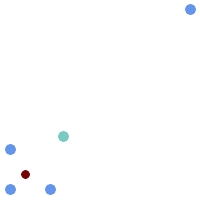## Name

ST_GeometricMedian — Returns the geometric median of a MultiPoint.

## Synopsis

`geometry ST_GeometricMedian (` geometry geom, float8 tolerance = NULL, int max_iter = 10000, boolean fail_if_not_converged = false`)`;

## Description

Computes the approximate geometric median of a MultiPoint geometry using the Weiszfeld algorithm. The geometric median is the point minimizing the sum of distances to the input points. It provides a centrality measure that is less sensitive to outlier points than the centroid (center of mass).

The algorithm iterates until the distance change between successive iterations is less than the supplied `tolerance` parameter. If this condition has not been met after `max_iterations` iterations, the function produces an error and exits, unless `fail_if_not_converged` is set to `false` (the default).

If a `tolerance` argument is not provided, the tolerance value is calculated based on the extent of the input geometry.

If present, the input point M values are interpreted as their relative weights.

Availability: 2.3.0

Enhanced: 2.5.0 Added support for M as weight of points.This function supports 3d and will not drop the z-index.This function supports M coordinates.

## ExamplesComparison of the centroid (turquoise point) and geometric median (red point) of a four-point MultiPoint (yellow points).

```WITH test AS (
SELECT 'MULTIPOINT((0 0), (1 1), (2 2), (200 200))'::geometry geom)
SELECT
ST_AsText(ST_Centroid(geom)) centroid,
ST_AsText(ST_GeometricMedian(geom)) median
FROM test;
centroid      |                 median
--------------------+----------------------------------------
POINT(50.75 50.75) | POINT(1.9761550281255 1.9761550281255)
(1 row)
```